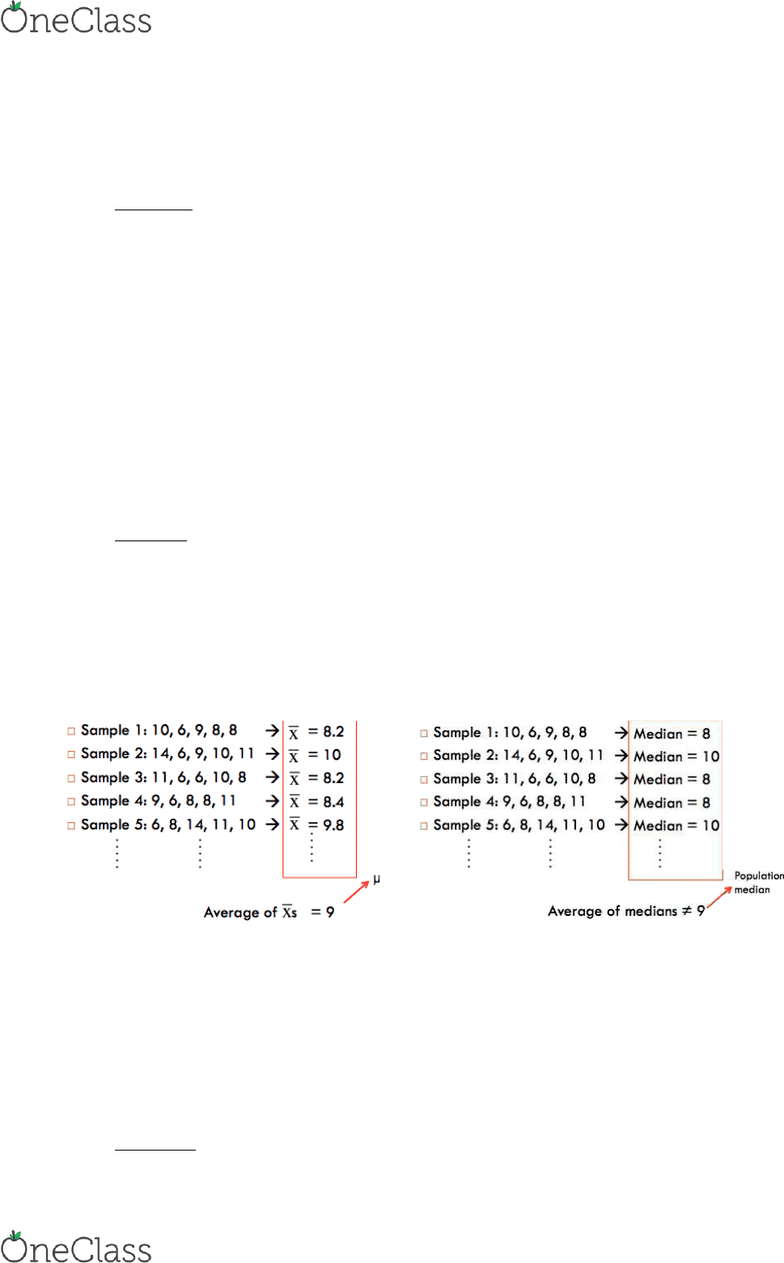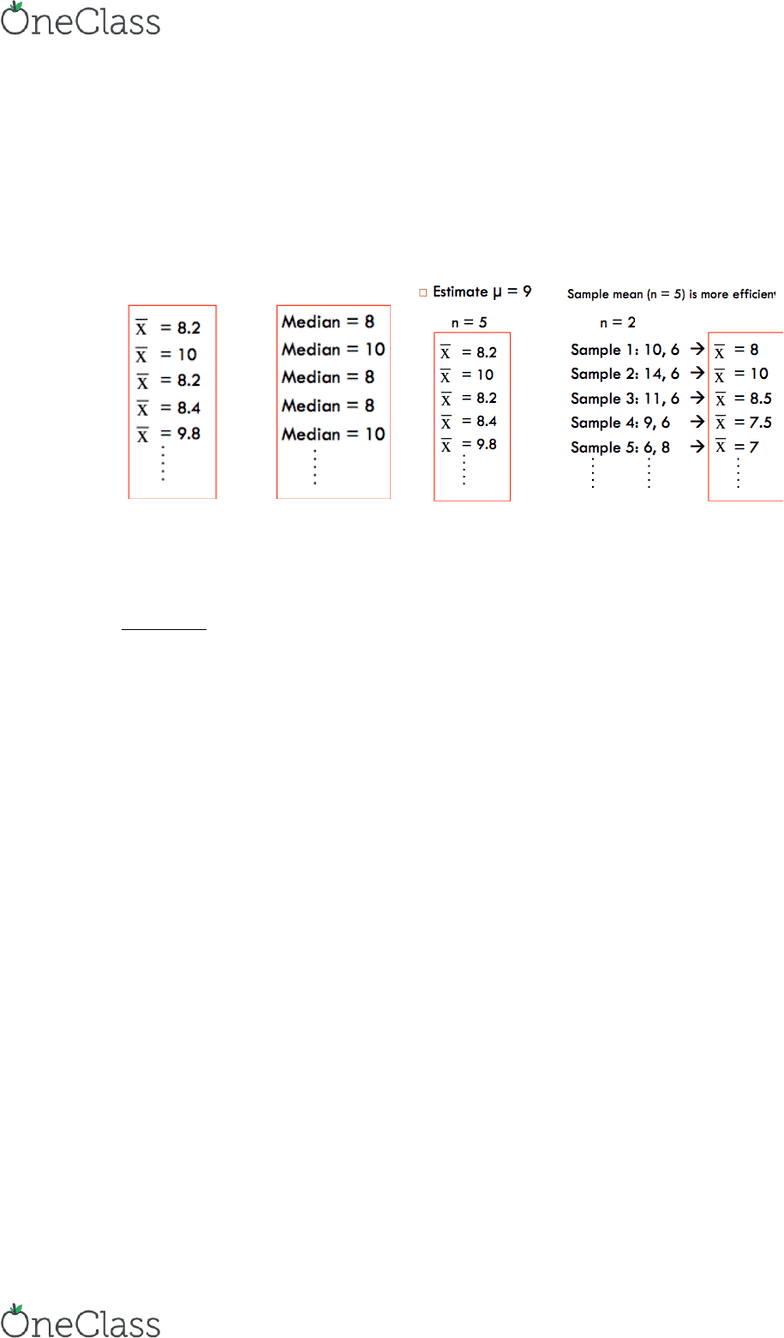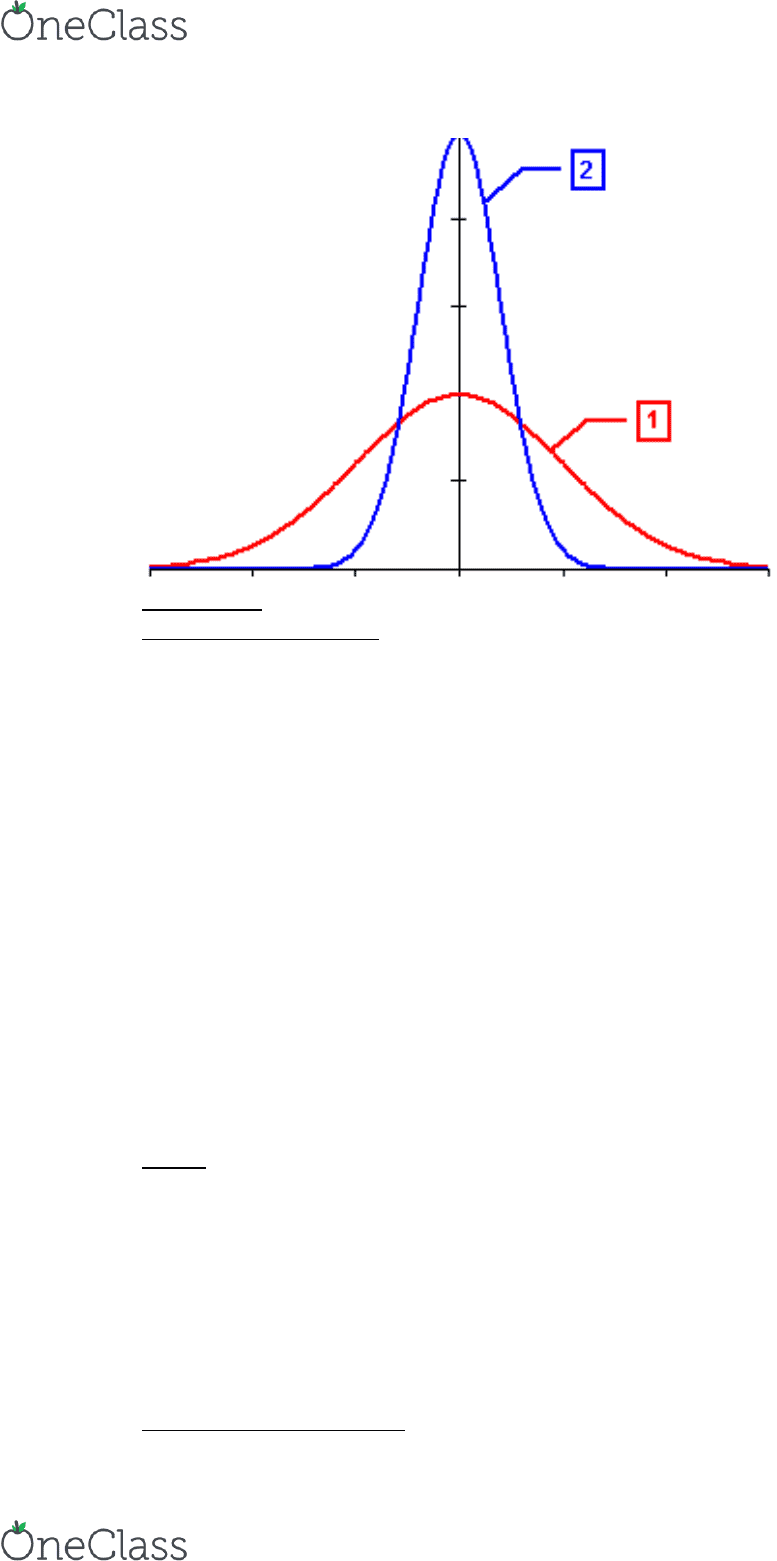Class Notes (1,100,000)
CA (650,000)
UTSC (30,000)
Psychology (9,000)
Lecture 4

# PSYB07H3 Lecture Notes - Lecture 4: Interquartile Range, Statistical Inference, Quartile

Department
Psychology
Course Code
PSYB07H3
Professor
Dwayne Pare
Lecture
4

This preview shows pages 1-3. to view the full 12 pages of the document.Lecture Notes September 28, 2016
Lecture #4 – PSYB07 – Descriptive Statistics II
Lecture #4 Descriptive Statistics II
Unbiased
Unbiased estimator
Expected value of =μ
o From an individual sample may not =μ (of a population)
sample may not equal population
oIf I average the from many samples, the average (mean of the means)
would = μ
Sample of 10 may or may not be a representation of the entire
class (population) – it depends
If you do a sample of 10, another sample of 10, another sample
of 10, then you get the average of these then average their
results, that is a better representation of the population and
therefore unbiased
oNot true for median nor mode
Example
Population = 6, 6, 8, 8, 9, 9, 10, 11, 14
µ (population mean) = 9
Median = 9
Collect a sample
à µ
Sample median à Population median
median
is
biased to the sample you take in the
picture on the right ^
Efficiency
1
find more resources at oneclass.com
find more resources at oneclass.com

Only pages 1-3 are available for preview. Some parts have been intentionally blurred.Lecture Notes September 28, 2016
Lecture #4 – PSYB07 – Descriptive Statistics II
Estimate µ (or population median) = 9
oSample means cluster more closely to 9
Because we know the data is all being used, every time we take
a sample, that sample is closer to 9 than the median may be
Mean is more efficient in this way
omean à More efficient
- Too get more efficiency: Take one sample with a large N – gives us a close
approximation to the population parameter
Summary
Mean:
o Resistant - gets pulled be outliers
o Sufficient – uses all of the data
o Unbiased – when you get a sample statistic the expected value is =
to population
o Efficient – the means efficient and that increases with the larger
sample
Inferential
oSample mean à Population mean
oThe requirement for these inferential statistics which is the
sample mean is inferring the population mean
oInferential statistics make predictions about a population from
observations and analyses of a sample (with the use of statistical tests)
oRather than doing multiple samples, we can just do a large sample and
we can figure out if our results are valued
2
find more resources at oneclass.com
find more resources at oneclass.com

Only pages 1-3 are available for preview. Some parts have been intentionally blurred.Lecture Notes September 28, 2016
Lecture #4 – PSYB07 – Descriptive Statistics II
Variability
Measures of Variability
oHow far is the rest of your data from the centre of the distribution?
Small variability (49&51) they are not very far away from the
middle
Mean of an exam =70, then we see the variability is between
(68-72)
oBlue graph = very little variability
oRed = lots of variability (data across the entire spectrum)
Range
oDistance between lowest and highest score
Interquartile Range (IQR)
oDistance between 1st and 3rd quartile
Variance & Standard Deviation (MOST IMPORTANT ASPECT OF
VARIABILITY BECAUSE IT IS A MEASURE THAT IS USED IN EVERYTHING
WE DO IN STATISTICS)
o“Average” distance from the mean
Range
Range = highest score – lowest score
Population = 6, 6, 8, 8, 9, 9, 10, 11, 14
oRange = 14 – 6 = 8
Low Resistance - sensitive to outliers
oLet’s say someone watched for 20 hours and someone watches for 3
hours… they are more extreme and therefore it would not be a good
representation of the true range
Interquartile Range (IQR)
3
find more resources at oneclass.com
find more resources at oneclass.com